Next: Bibliography

# FUZZY RULES AND REGULARIZATION THEORY

Jörg C. Lemm
Institut für Theoretische Physik I
Wilhelm-Klemm-Str.9, D-48149 Münster, Germany
Tel. (+49)251/83-34922
Fax (+49)251/83-36328
lemm@uni-muenster.de

ABSTRACT: Regularization theory and stochastic processes have the advantage that a-priori information is expressed directly in terms of the function values of interest. Classical regularization functionals are convex and possess a unique minimum. On the other hand, convex functionals can only implement AND-like combinations of approximation conditions. The paper discusses the empirical measurement of general, also non-convex a-priori information and its technical implementation by fuzzy logical-like combinations of quadratic building blocks.

1. INTRODUCTION

The generalization ability of empirical learning systems is essentially based on dependencies between known training data and future test situations. Those dependencies are part of the a-priori information necessary for any empirical learning. We discuss in this paper aspects of the empirical measurement or control of a-priori information.

We concentrate on the regularization approach (Tikhonov, 1963). Given training data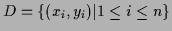we aim in finding an approximation or hypothesis function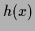which minimizes a given error functional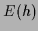. A typical functional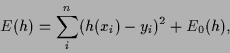(1)

consists of two terms, a mean-square training error and an additional prior term. A possible smoothness related prior term is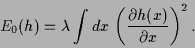(2)

In regularization theory prior terms, and hence the dependencies between training and test data, are directly formulated in terms of the function values. Often this is much easier to interpret than dependencies which are implicit in a chosen parameterization of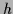(for example as neural network) or in a chosen algorithm (for example with specific initial conditions or stopping rule). From a Bayesian point of view regularization theory has an interpretation in terms of stochastic processes (Doob, 1953, Wahba, 1990) or stochastic fields like they appear in statistical mechanics or statistical field theory (Itzykson & Drouffe, 1989). Classical regularization approaches include for example Radial Basis Functions approaches and various spline methods(Poggio & Girosi, 1990, Girosi, Jones, & Poggio, 1995).

In Section 2 we discuss aspects of empirical control of prior knowledge. Section 3 shows non-convex functionals obtained by fuzzy-like methods.

2. EMPIRICAL MEASUREMENT OF A-PRIORI INFORMATION

The crucial role of the dependencies between training data and test data for generalization would in principle require strict empirical control of these dependencies. Such an empirical control or measurement of a-priori information is of special practical importance in low data scenarios or when security aspects require to control the response to all potential test situations. Three reasons may be mainly responsible for the lack of empirical measurement of a-priori information in practice:

1.
A-priori information corresponds to an infinite number of data. Empirical measurement of a-priori information requires data for every potential test situation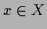. For infinite or even continuous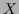this corresponds to an infinite amount of data. A simple example are bounds like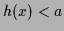,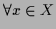. The practical problem is: How can an infinite number of data be measured in practice?
2.
A-priori information is often complex, unsharp, and non-convex. The dependency structure may be very complicated. For example, a class of objects, like faces, cars, phonemes, pedestrians, characters,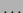, is never well defined by the available training examples alone. It is implicitly defined by the selection process of training examples, often done by human experts. Hence, mostly only qualitative, e.g. verbal, information will be available. Prior knowledge enumerating alternative possibilities has a non-convex structure. For example, one may expect an eye to be open OR closed, or a photo to show either the seashore OR mountains. Such OR-like combinations yield regularization functionals with multiple minima and non-linear stationarity equations.
3.
Explicit implementation of prior knowlegde is computationally costly. Typically, parameterized function spaces, e.g. neural networks, contain dependencies which are difficult to analyze and to control by humans. To avoid implicit restrictions introduced by parameterizations one has to treat the single function valuesitself as primary degrees of freedom. Such approaches, like regularization theory, stochastic processes or statistical field theory in physics require usually large computational resources.

The problems can be attacked at least partly.

1.
A-priori information can be measured by a-posteriori control. Bounds for an infinite number of function values, for example, can simply be ensured by using measurement devices with a cut-off function. Analogously, exact or approximate symmetries, like smoothness for infinitesimal translations, can be realized by input noise or input averaging with respect to the symmetry group under consideration (Bishop, 1995, Leen, 1995, and Lemm, 1996). The key point is that even for a formally infinite number of potential test situationsonly a finite number of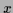actually appears as test situation. Hence, a-priori information corresponding to an infinite number of data must be enforced by a-posteriori control of application situation.
2.
Unsharp prior knowledge can be quantified using fuzzy techniques. As most practical important functions or object classes are not sufficiently well defined by training examples alone, but by training example selecting and performance evaluating human experts, there is a clear need in quantifying unsharp, verbally formulated knowledge. (See for example Klir & Yuan, 1995, 1996.) For example, verbal descriptions of faces can be used to construct quantitative templates for prototypical forms of constituents (e.g. eyes, nose, mouth), their variants (e.g. open vs. closed mouth, translated, scaled, or deformed eyes) and relations (e.g. typical spatial distances). Defining AND and OR-like combinations of constituent templates the image of a face, incomplete and disturbed by noise, may be reconstructed by approximating given noisy data points of the incomplete image AND typical templates of constituents AND their spatial relations in either one of their variants, for example, a template for open OR for closed eyes.
3.
Increasing computational resources allow the use of more costly numerical methods. Especially low dimensional problems are suited for adaption of methods of statistical mechanics or lattice field theory(Montvay & Muenster, 1994). Typical examples are Monte-Carlo methods used for one-dimensional time series or two-dimensional image reconstruction tasks (Zhu & Mumford,1997).

3. NON-CONVEX FUNCTIONALS

We choose quadratic building blocks to construct arbitrary complex prior functionals. Classical regularization functionals like (1) consist of a sum of quadratic terms. Indeed, we may write for (2)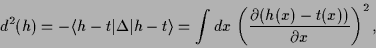(3)

where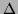denotes the Laplacian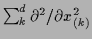(for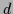-dimensional) and we used partial integration assuming vanishing boundary terms and angular brackets to denote the matrix elements of a symmetric operator. In (3) a template function''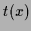has been introduced. This functionis identically zero in (2) but comparing with a mean-square training error term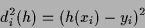(4)

we see thatin (3) generalizes discrete training data to continuous data. Such continuous data functions are always present in quadratic prior terms but can be chosen identically zero in functionals of form (1), where only one quadratic prior term is present, by solving for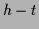instead for. For functionals with more than one prior term not all template functions can be set to zero at the same time. Hence, we include explicitly continuous data functionsand use as fundamental building blocks quadratic concepts'' of the form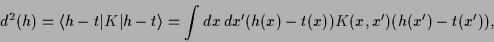(5)

where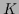is a positive definite real symmetric matrix or operator, so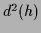represents a square distance. Quadratic concepts encompass the mean-square error as well as classical prior terms and correspond to a Gaussian process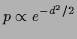with covariance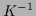.

A sum of terms as in (1) implements an AND in probabilistic interpretation, i.e., functional (1) requires training data AND prior term to be approximated. A probabilistic OR can be implemented by a mixture model. In the case of two alternative concepts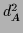and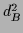with equal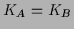in addition to standard training data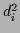one can minimize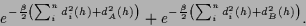(6)

Here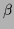is a technically useful convexity parameter also known as inverse temperature (Rose, Gurewitz, & Fox, 1990, Yuille & Kosowski, 1994). Non-zero template functionsyield inhomogeneous stationarity equations which are, for example, similar to equations appearing in quantum mechanical scattering theory (Lemm, 1995). Alternatively, a product implementation of OR for square distances yields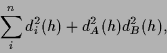(7)

resembling the Landau-Ginzburg treatment of phase transitions in statistical mechanics. For technical details see (Lemm, 1996).

4. CONCLUSIONS

The paper emphasizes the possibility of empirical measurement or control of a-priori information corresponding to an infinite number of data. Methods have been presented implementing complex prior knowledge by combinations of quadratic building blocks. OR-like combinations resulting in non-convex regularization functionals require methods going beyond classical quadratic regularization or Gaussian process approaches.

ACKNOWLEDGEMENTS

The author was supported by a Postdoctoral Fellowship (Le 1014/1-1) from the Deutsche Forschungsgemeinschaft and a NSF/CISE Postdoctoral Fellowship at the Massachusetts Institute of Technology. He also wants to thank Federico Girosi and Tomaso Poggio for stimulating discussions.Next: Bibliography
Joerg_Lemm 2000-09-22